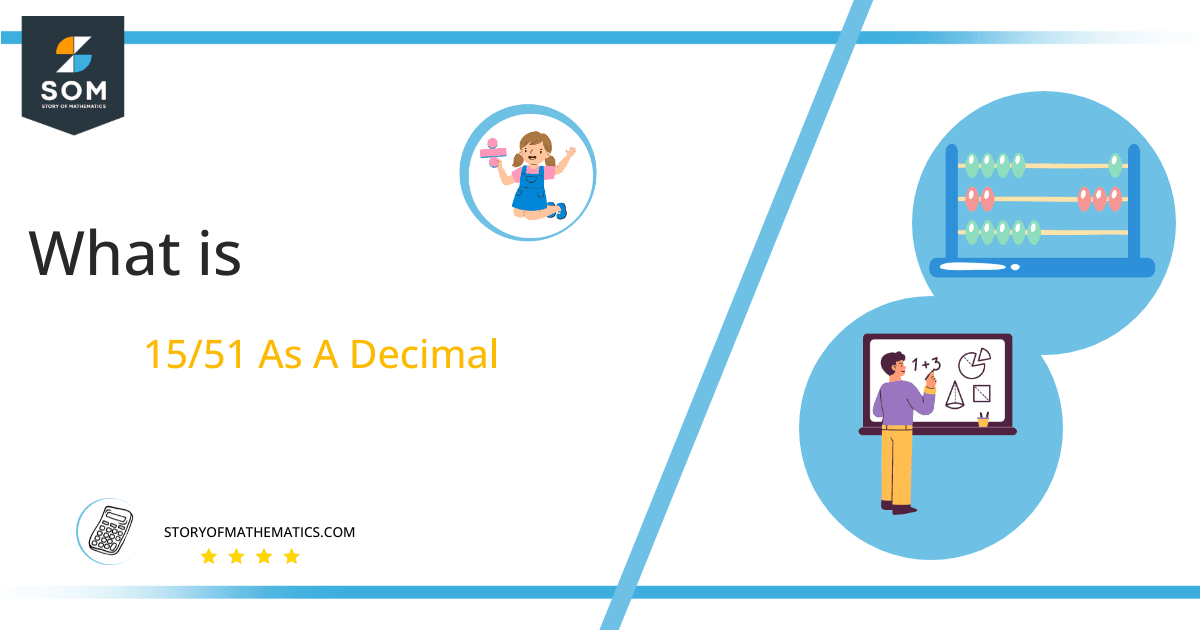# What Is 15/51 as a Decimal + Solution With Free Steps

The fraction 15/51 as a decimal is equal to 0.29411765.

The division is one of the most challenging mathematical operations because fractions require it. But by employing the later-discussed strategy, we can simplify it. The p/q form, where and are referred to as the Numerator and Denominator, can be used to represent a Fraction.Here, we are more interested in the division types that result in a Decimal value, as this can be expressed as a Fraction. We see fractions as a way of showing two numbers having the operation of Division between them that result in a value that lies between two Integers.

Now, we introduce the method used to solve said fraction-to-decimal conversion, called Long Division, which we will discuss in detail moving forward. So, let’s go through the Solution of fraction 15/51.

## Solution

First, we convert the fraction components, i.e., the numerator and the denominator, and transform them into the division constituents, i.e., the Dividend and the Divisor, respectively.

This can be done as follows:

Dividend = 15

Divisor = 51

We introduce the most important quantity in our division process: the Quotient. The value represents the Solution to our division and can be expressed as having the following relationship with the Division constituents:

Quotient = Dividend $\div$ Divisor = 15 $\div$ 51

This is when we go through the Long Division solution to our problem. The following figure shows the long division:Figure 1

## 15/51 Long Division Method

We start solving a problem using the Long Division Method by first taking apart the division’s components and comparing them. As we have 15 and 51, we can see how 15 is Smaller than 51, and to solve this division, we require that 15 be Bigger than 51.

This is done by multiplying the dividend by 10 and checking whether it is bigger than the divisor or not. If so, we calculate the Multiple of the divisor closest to the dividend and subtract it from the Dividend. This produces the Remainder, which we then use as the dividend later.

Now, we begin solving for our dividend 15, which after getting multiplied by 10 becomes 150.

We take this 150 and divide it by 51; this can be done as follows:

150 $\div$ 51 $\approx$ 2

Where:

51 x 2 = 102

This will lead to the generation of a Remainder equal to 150 – 102 = 48. Now this means we have to repeat the process by Converting the 48 into 480 and solving for that:

480 $\div$ 51 $\approx$ 9

Where:

51 x 9 = 459

This, therefore, produces another Remainder equal to 480 – 459= 21. Now we must solve this problem to the Third Decimal Place for accuracy, so we repeat the process with dividend 210.

210 $\div$ 51 $\approx$ 4

Where:

51 x 4 = 204

Finally, we have a Quotient generated after combining the three pieces of it as 0.294=z, with a Remainder equal to 6.Images/mathematical drawings are created with GeoGebra.